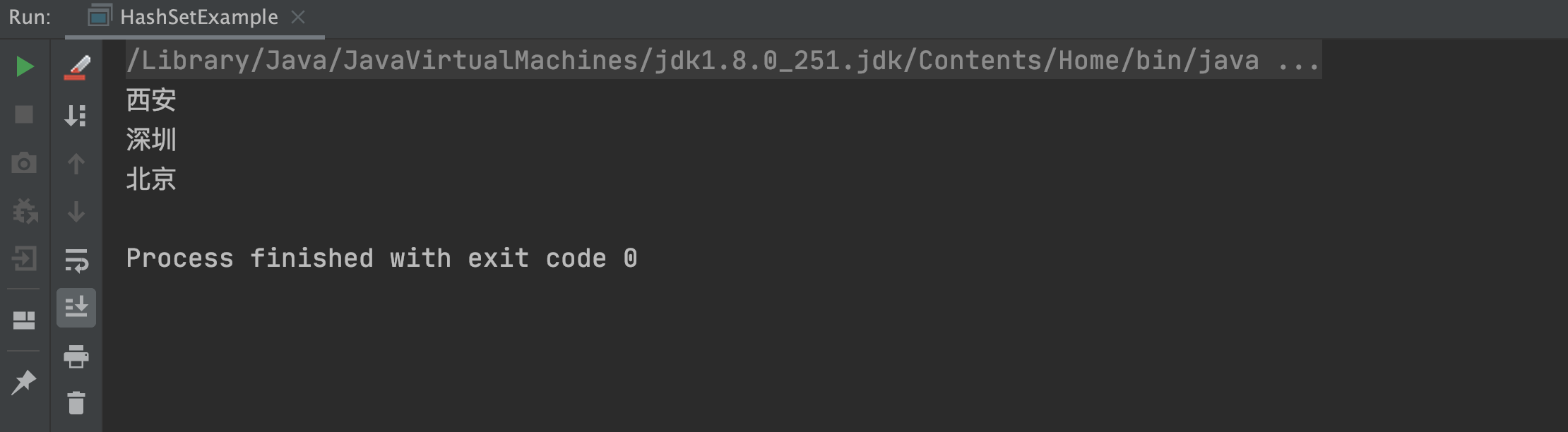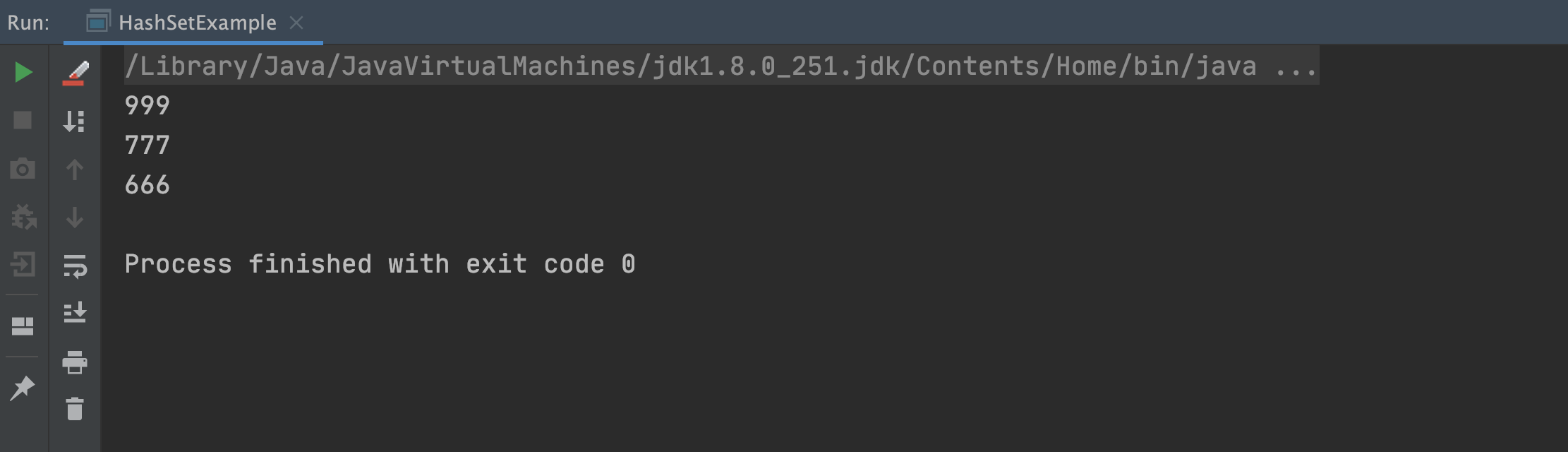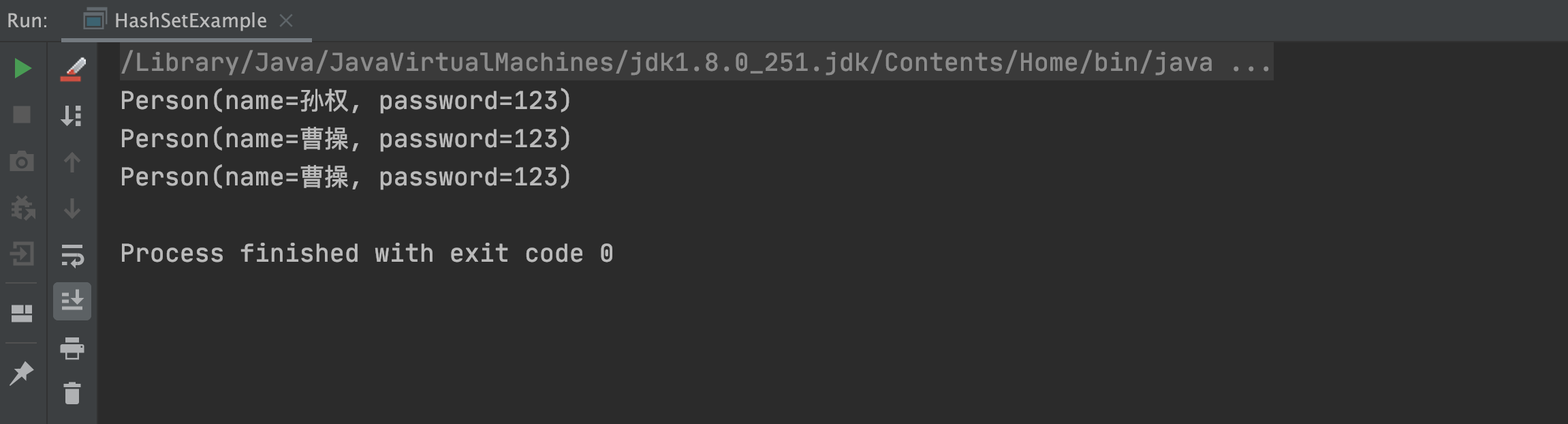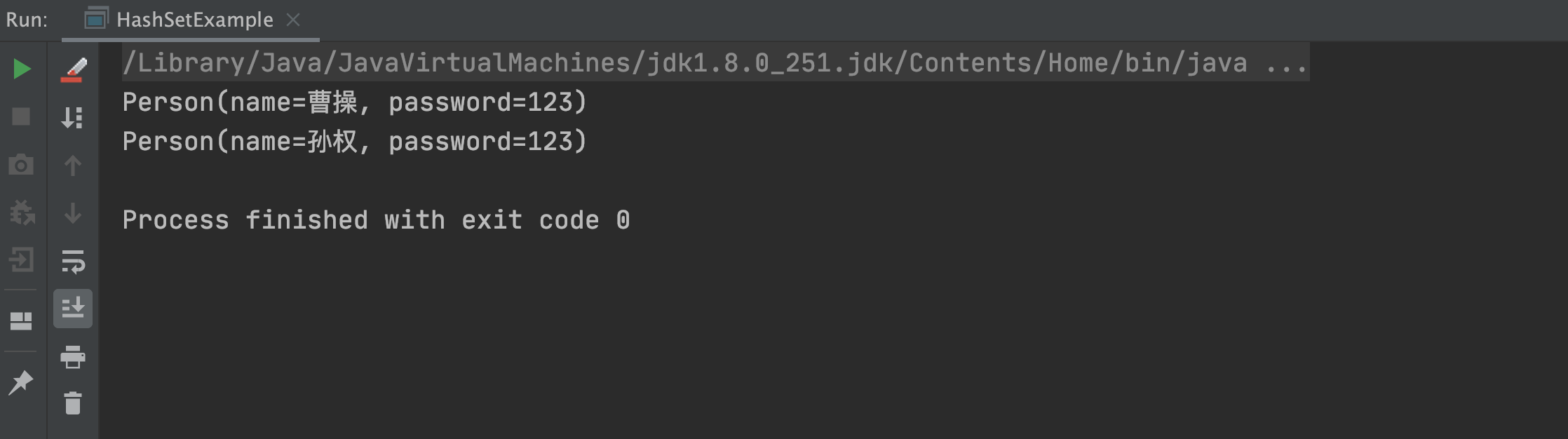# 面试官：HashSet 如何保证元素不重复？

• 2021 年 12 月 21 日
• 本文字数：4625 字

阅读完需：约 15 分钟HashSet 实现了 Set 接口，由哈希表（实际是 HashMap）提供支持。HashSet 不保证集合的迭代顺序，但允许插入 null 值。也就是说 HashSet 不能保证元素插入顺序和迭代顺序相同。HashSet 具备去重的特性，也就是说它可以将集合中的重复元素自动过滤掉，保证存储在 HashSet 中的元素都是唯一的。

## 1.HashSet 基本用法

// 创建 HashSet 集合HashSet<String> strSet = new HashSet<>();// 给 HashSet 添加数据strSet.add("Java");strSet.add("MySQL");strSet.add("Redis");// 循环打印 HashSet 中的所有元素strSet.forEach(s -> System.out.println(s));

## 2.HashSet 无序性

HashSet 不能保证插入元素的顺序和循环输出元素的顺序一定相同，也就是说 HashSet 其实是无序的集合，具体代码示例如下：

HashSet<String> mapSet = new HashSet<>();mapSet.add("深圳");mapSet.add("北京");mapSet.add("西安");// 循环打印 HashSet 中的所有元素mapSet.forEach(m -> System.out.println(m));## 3.HashSet 错误用法

### 3.1 HashSet 与基本数据类型

HashSet<Long> longSet = new HashSet<>();longSet.add(666l);longSet.add(777l);longSet.add(999l);longSet.add(666l);// 循环打印 HashSet 中的所有元素longSet.forEach(l -> System.out.println(l));### 3.2 HashSet 与自定义对象类型

public class HashSetExample {    public static void main(String[] args) {        HashSet<Person> personSet = new HashSet<>();        personSet.add(new Person("曹操", "123"));        personSet.add(new Person("孙权", "123"));        personSet.add(new Person("曹操", "123"));        // 循环打印 HashSet 中的所有元素        personSet.forEach(p -> System.out.println(p));    }}@Getter@Setter@ToStringclass Person {    private String name;    private String password;    public Person(String name, String password) {        this.name = name;        this.password = password;    }}@Overridepublic int hashCode() {    return Long.hashCode(value);}public boolean equals(Object obj) {    if (obj instanceof Long) {        return value == ((Long)obj).longValue();    }    return false;}//省略其他源码......

@Setter@Getter@ToStringclass Person {    private String name;    private String password;    public Person(String name, String password) {        this.name = name;        this.password = password;    }    @Override    public boolean equals(Object o) {        if (this == o) return true; // 引用相等返回 true        // 如果等于 null，或者对象类型不同返回 false        if (o == null || getClass() != o.getClass()) return false;        // 强转为自定义 Person 类型        Person persion = (Person) o;        // 如果 name 和 password 都相等，就返回 true        return Objects.equals(name, persion.name) &&                Objects.equals(password, persion.password);    }    @Override    public int hashCode() {        // 对比 name 和 password 是否相等        return Objects.hash(name, password);    }}## 4.HashSet 如何保证元素不重复？

// hashmap 中 put() 返回 null 时，表示操作成功public boolean add(E e) {    return map.put(e, PRESENT)==null;}

// 返回值：如果插入位置没有元素则返回 null，否则返回上一个元素public V put(K key, V value) {    return putVal(hash(key), key, value, false, true);}

    final V putVal(int hash, K key, V value, boolean onlyIfAbsent,                   boolean evict) {        Node<K, V>[] tab;        Node<K, V> p;        int n, i;        //如果哈希表为空，调用 resize() 创建一个哈希表，并用变量 n 记录哈希表长度        if ((tab = table) == null || (n = tab.length) == 0)            n = (tab = resize()).length;        /**         * 如果指定参数 hash 在表中没有对应的桶，即为没有碰撞         * Hash函数，(n - 1) & hash 计算 key 将被放置的槽位         * (n - 1) & hash 本质上是 hash % n 位运算更快         */        if ((p = tab[i = (n - 1) & hash]) == null)            // 直接将键值对插入到 map 中即可            tab[i] = newNode(hash, key, value, null);        else {// 桶中已经存在元素            Node<K, V> e;            K k;            // 比较桶中第一个元素(数组中的结点)的 hash 值相等，key 相等            if (p.hash == hash &&                    ((k = p.key) == key || (key != null && key.equals(k))))                // 将第一个元素赋值给 e，用 e 来记录                e = p;                // 当前桶中无该键值对，且桶是红黑树结构，按照红黑树结构插入            else if (p instanceof TreeNode)                e = ((TreeNode<K, V>) p).putTreeVal(this, tab, hash, key, value);                // 当前桶中无该键值对，且桶是链表结构，按照链表结构插入到尾部            else {                for (int binCount = 0; ; ++binCount) {                    // 遍历到链表尾部                    if ((e = p.next) == null) {                        p.next = newNode(hash, key, value, null);                        // 检查链表长度是否达到阈值，达到将该槽位节点组织形式转为红黑树                        if (binCount >= TREEIFY_THRESHOLD - 1) // -1 for 1st                            treeifyBin(tab, hash);                        break;                    }                    // 链表节点的<key, value>与 put 操作<key, value>                    // 相同时，不做重复操作，跳出循环                    if (e.hash == hash &&                            ((k = e.key) == key || (key != null && key.equals(k))))                        break;                    p = e;                }            }            // 找到或新建一个 key 和 hashCode 与插入元素相等的键值对，进行 put 操作            if (e != null) { // existing mapping for key                // 记录 e 的 value                V oldValue = e.value;                /**                 * onlyIfAbsent 为 false 或旧值为 null 时，允许替换旧值                 * 否则无需替换                 */                if (!onlyIfAbsent || oldValue == null)                    e.value = value;                // 访问后回调                afterNodeAccess(e);                // 返回旧值                return oldValue;            }        }        // 更新结构化修改信息        ++modCount;        // 键值对数目超过阈值时，进行 rehash        if (++size > threshold)            resize();        // 插入后回调        afterNodeInsertion(evict);        return null;    }

## 总结

HashSet 底层是由 HashMap 实现的，它可以实现重复元素的去重功能，如果存储的是自定义对象必须重写 hashCode 和 equals 方法。HashSet 保证元素不重复是利用 HashMap 的 put 方法实现的，在存储之前先根据 key 的 hashCode 和 equals 判断是否已存在，如果存在就不在重复插入了，这样就保证了元素的不重复。## 评论